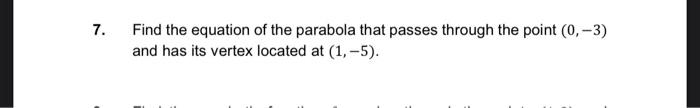Home / Expert Answers / Precalculus / find-the-equation-of-the-parabola-that-passes-through-the-point-0-3-and-has-its-vertex-located-pa878

# (Solved): Find the equation of the parabola that passes through the point (0,3) and has its vertex located ...Find the equation of the parabola that passes through the point and has its vertex located at .

We have an Answer from Expert